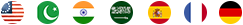adad meaning in english is Number. adad in Urdu is written as عدد find similar meaning of adad with translation as Act, Amount, Bit, Come, Count, Enumerate, Figure, Issue, List, Numeral, Numerate, Routine, Total, Turn

 عدد ہندسہ مقدار

### NUMBER Synonyms & Definition

• Number Meaning In English
1. (n.) Numerousness; multitude.
2. (n.) Quantity, regarded as made up of an aggregate of separate things.
3. (n.) That which admits of being counted or reckoned; a unit, or an aggregate of units; a numerable aggregate or collection of individuals; an assemblage made up of distinct things expressible by figures.
4. (n.) That which is regulated by count; poetic measure, as divisions of time or number of syllables; hence, poetry, verse; -- chiefly used in the plural.
5. (n.) To amount; to equal in number; to contain; to consist of; as, the army numbers fifty thousand.
6. (n.) To count; to reckon; to ascertain the units of; to enumerate.
7. (n.) A numeral; a word or character denoting a number; as, to put a number on a door.
8. (n.) A collection of many individuals; a numerous assemblage; a multitude; many.
9. (n.) The measure of the relation between quantities or things of the same kind; that abstract species of quantity which is capable of being expressed by figures; numerical value.
10. (n.) The state or quality of being numerable or countable.
11. (n.) To give or apply a number or numbers to; to assign the place of in a series by order of number; to designate the place of by a number or numeral; as, to number the houses in a street, or the apartments in a building.
12. (n.) The distinction of objects, as one, or more than one (in some languages, as one, or two, or more than two), expressed (usually) by a difference in the form of a word; thus, the singular number and the plural number are the names of the forms of a word indicating the objects denoted or referred to by the word as one, or as more than one.
13. (n.) To reckon as one of a collection or multitude.
14. (n.) That which admits of being counted or reckoned; a unit, or an aggregate of units; a numerable aggregate or collection of individuals; an assemblage made up of distinct things expressible by figures.
15. (n.) To reckon as one of a collection or multitude.
16. (n.) To count; to reckon; to ascertain the units of; to enumerate.
17. (n.) The distinction of objects, as one, or more than one (in some languages, as one, or two, or more than two), expressed (usually) by a difference in the form of a word; thus, the singular number and the plural number are the names of the forms of a word indicating the objects denoted or referred to by the word as one, or as more than one.
18. (n.) The measure of the relation between quantities or things of the same kind; that abstract species of quantity which is capable of being expressed by figures; numerical value.
19. (n.) That which is regulated by count; poetic measure, as divisions of time or number of syllables; hence, poetry, verse; -- chiefly used in the plural.
20. (n.) A numeral; a word or character denoting a number; as, to put a number on a door.
21. (n.) To give or apply a number or numbers to; to assign the place of in a series by order of number; to designate the place of by a number or numeral; as, to number the houses in a street, or the apartments in a building.
22. (n.) Quantity, regarded as made up of an aggregate of separate things.
23. (n.) Numerousness; multitude.
24. (n.) The state or quality of being numerable or countable.
25. (n.) To amount; to equal in number; to contain; to consist of; as, the army numbers fifty thousand.
26. (n.) A collection of many individuals; a numerous assemblage; a multitude; many.

Synonyms:
Act, Amount, Bit, Come, Count, Enumerate, Figure, Issue, List, Numeral, Numerate, Routine, Total, Turn,

## MULTI LANGUAGE DICTIONARYFROM: English Urdu Arabic Hindi Spanish French German
 TO: Urdu Arabic Hindi Spanish French German English